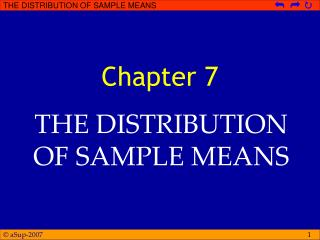DownloadDownload PresentationChapter 7

# Chapter 7

Download Presentation## Chapter 7

- - - - - - - - - - - - - - - - - - - - - - - - - - - E N D - - - - - - - - - - - - - - - - - - - - - - - - - - -
##### Presentation Transcript

1. Chapter 7 THE DISTRIBUTION OF SAMPLE MEANS

2. Imagine an urn filled with balls… • Two-third of the balls are one color, and the remaining one-third are a second color • One individual select 5 balls from the urn and finds that 4 are red and 1 is white • Another individual select 20 balls and finds that 12 are red and 8 are white • Which of these two individuals should feel more confident that the urn contains two third red balls and one-third white balls than the opposite?

3. The CORRECT ANSWER • The larger sample gives a much stronger justification for concluding that the balls in the urn predominantly red • With a small number, you risk obtaining an unrepresentative sample • The larger sample is much more likely to provide an accurate representation of the population • This is an example of the law of large number which states that large samples will be representative of the population from which they are selected

4. OVERVIEW • Whenever a score is selected from a population, you should be able to compute a z-score • And, if the population is normal, you should be able to determine the probability value for obtaining any individual score • In a normal distribution, a z-score of +2.00 correspond to an extreme score out in the tail of the distribution, and a score at least large has a probability of only p = .0228

5. OVERVIEW • In this chapter we will extend the concepts of z-scores and probability to cover situation with larger samples • We will introduce a procedure for transforming a sample mean into a z-score

6. THE DISTRIBUTION OF SAMPLE MEANS • Two separate samples probably will be different even though they are taken from the same population • The sample will have different individual, different scores, different means, and so on • The distribution of sample means is the collection of sample means for all the possible random samples of a particular size (n) that can be obtained from a population

7. COMBINATION n! • Consider a population that consist of 5 scores: 3, 4, 5, 6, and 7 • Mean population = ? • Construct the distribution of sample means for n = 1, n = 2, n = 3, n = 4, n = 5 nCr = r! (n-r)!

8. SAMPLING DISTRIBUTION • … is a distribution of statistics obtained by selecting all the possible samples of a specific size from a population CENTRAL LIMIT THEOREM • For any population with mean μ and standard deviation σ, the distribution of sample means for sample size n will have a mean of μ and a standard deviation of σ/√n and will approach a normal distribution as n approaches infinity

9. The STANDARD ERROR OF MEAN • The value we will be working with is the standard deviation for the distribution of sample means, and it called the σM • Remember the sampling error • There typically will be some error between the sample and the population • The σM measures exactly how much difference should be expected on average between sample mean M and the population mean μ

10. The MAGNITUDE of THE σM • Determined by two factors: • The size of the sample, and • The standard deviation of the population from which the sample is selected

11. LEARNING CHECK • A population of scores is normal withμ = 100andσ = 15 • Describe the distribution of sample means for samples size n = 25 and n =100 • Under what circumstances will the distribution of samples means be a normal shaped distribution?

12. PROBABILITY AND THE DISTRIBUTION OF SAMPLE MEANS • The primary use of the standard distribution of sample means is to find the probability associated with any specific sample • Because the distribution of sample means present the entire set of all possible Ms, we can use proportions of this distribution to determine probabilities

13. EXAMPLE • The population of scores on the SAT forms a normal distribution with μ = 500andσ = 100. If you take a random sample of n = 16 students, what is the probability that sample mean will be greater that M = 540? σ M - μ σM = z = = 25 = 1.6 σM √n z = 1.6  Area C  p = .0548

14. LEARNING CHECK • The population of scores on the SAT forms a normal distribution with μ = 500andσ = 100. We are going to determine the exact range of values that is expected for sample mean 95% of the time for sample of n = 25 students See Example 7.3 on Gravetter’s book page 207